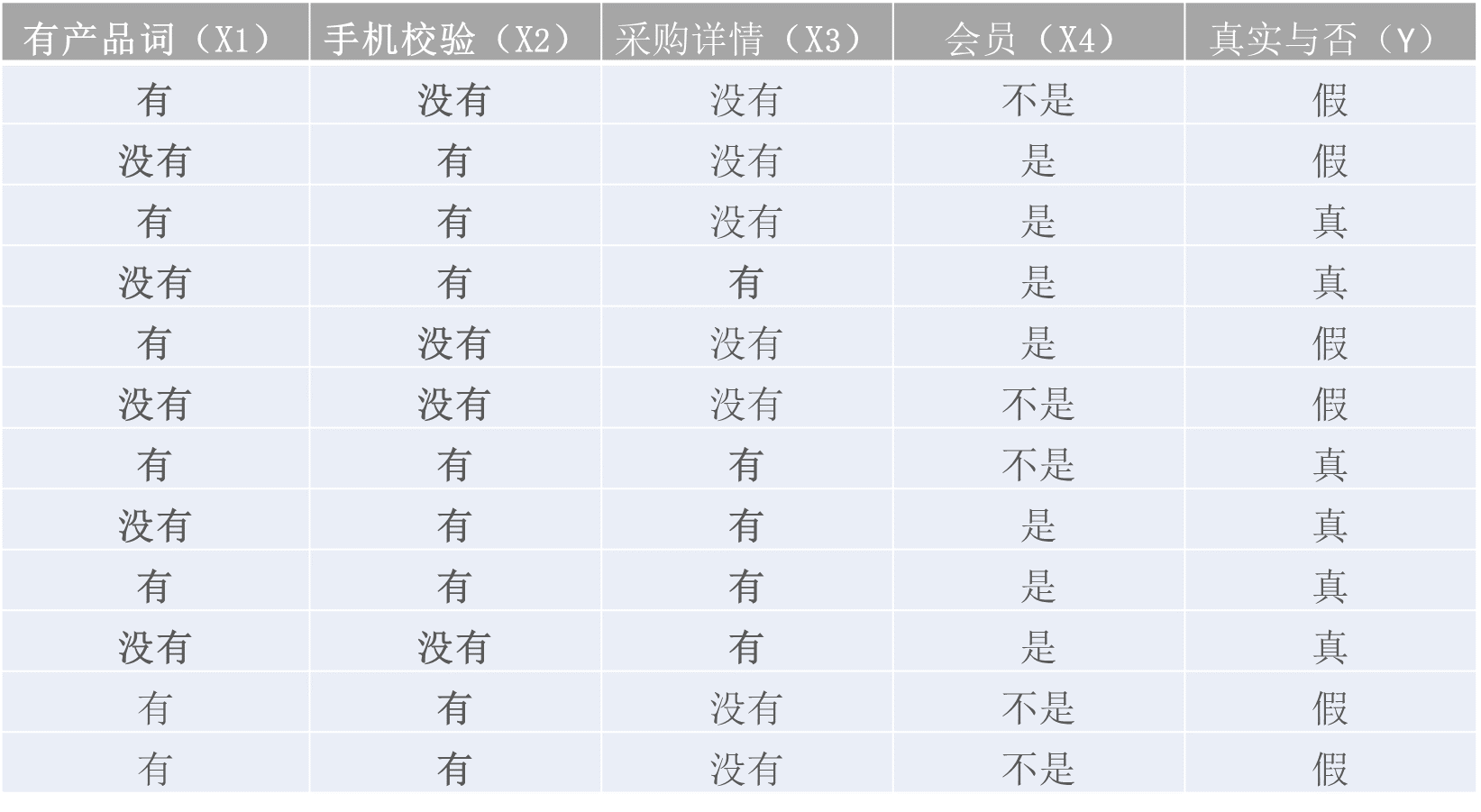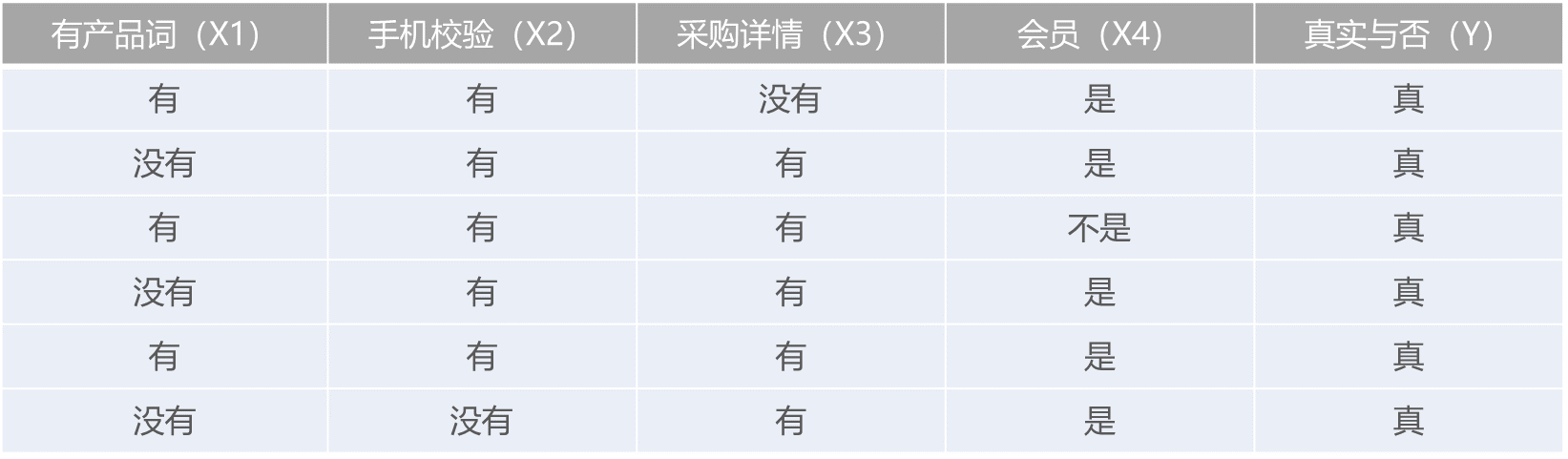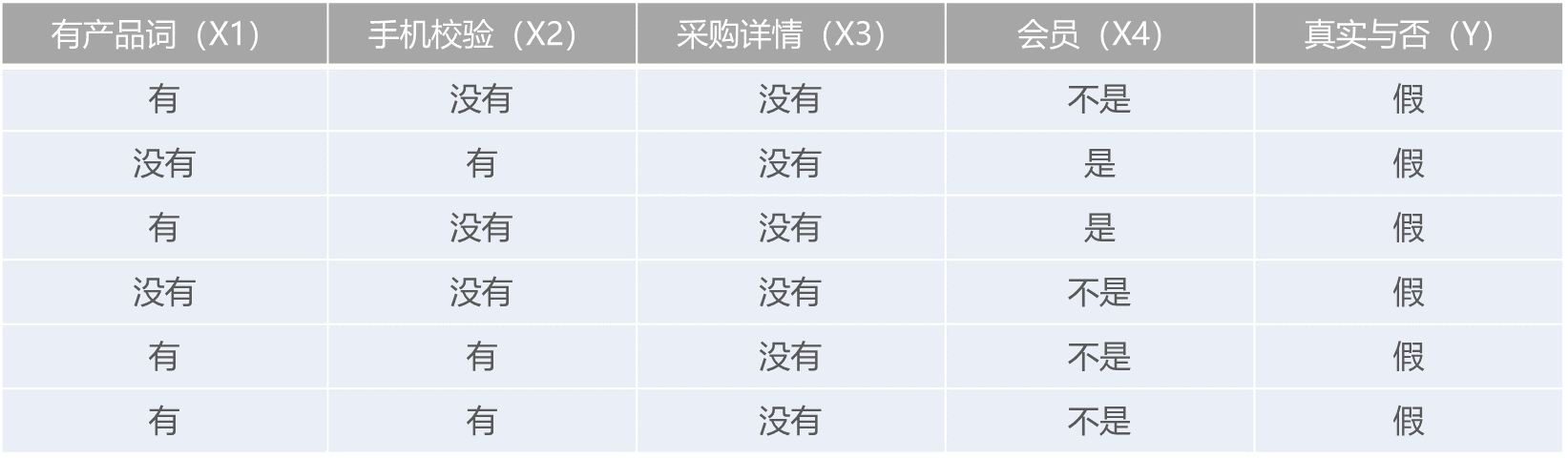APP## 机器学习 | 贝叶斯算法及应用## 基于条件概率的贝叶斯定律数学公式## 朴素贝叶斯算法

### 定义### 区别

KNN分类算法和决策树分类算法最终都是预测出实例的确定的分类结果，但是，有时候分类器会产生错误结果；而朴素贝叶斯分类算法则是给出一个最优的猜测结果，同时给出猜测的概率估计值。

### 推导

（1）特征属性X之间是独立的，所以得到（2）优化得（3）因为分母对于所有类别为常数，因为我们只要将分子最大化皆可。又因为各特征属性是条件独立的，所以有### 流程

• 设x={a1,a2,……,am}为待分类项，其中a为x的一个特征属性；
• 类别集合为C={y1,y2,……,yn}；
• 分别计算P(y1|x),P(y2|x),…….,P(yn|x)的值（贝叶斯公式）## 高斯朴素贝叶斯

### 定义

Gaussian Naive Bayes是指当特征属性为连续值时，而且分布服从高斯分布，那么在计算P(x|y)的时候可以直接使用高斯分布的概率公式，其他的与朴素贝叶斯一致## 伯努利朴素贝叶斯

### 定义

Bernoulli Naive Bayes是指当特征属性为连续值时，而且分布服从伯努利分布，  那么在计算P(x|y)的时候可以直接使用伯努利分布的概率公式：## 多项式朴素贝叶斯

### 定义

Multinomial Naive Bayes是指当特征属性服从多项分布(特征是离散的形式的时候)，从而，对于每个类别y，每个特征属性都有一个对应的参数  θy=(θy1,θy2,……,θyn)，其中n为特征属性的取值数目，那么P(xk=i|y)的概率为θyi。## 举例应用

### 准备阶段

a1  发布的采购信息产品词是否在我们词库中；

a2  发布信息时是否对手机号进行的短信验证码的校验；

a3  发布的采购信息是否有详情；

a4  发布者是不是网站的会员；

y1 线索为真实采购；

y2 线索为虚假采购；### 训练阶段

（1）对每个类别计算P（y）1. P(y=真实)=6/12（总样本数）=1/22. P(y=假)=6/12（总样本数）=1/2

（2）对每个特征属性计算所有划分的条件概率P(x | y )P(x1=有产品词 | y)=1/2

P(x1=没有产品词 | y)=1/2

P(x2=经过校验 | y)=5/6

P(x2=不经过校验 | y)=1/6

P(x3=有详情 | y)=5/6

P(x3=无详情 | y)=1/6

P(x4=会员 | y)=5/6

P(x4=非会员 | y)=1/6P(x1=有产品词 | y)=2/3

P(x1=没有产品词 | y)=1/3

P(x2=经过校验 | y)=1/2

P(x2=不经过校验 | y)=1/2

P(x3=有详情 | y)=0

P(x3=无详情 | y)=1

P(x4=会员 | y)=1/3

P(x4=非会员 | y)=2/3

### 应用阶段

（1）计算此条线索为真实的概率

P(xi | y=真实)= P(x1=没有产品词 | y)* P(x2=不经过校验 | y)* P(x3=无详情 | y)* P(x4=非会员 | y)

=1/2*1/6*1/6*1/6

P(y=真实)= 1/2（2）计算此条线索为虚假的概率

P(xi | y=虚假)= P(x1=无产品词 | y)* P(x2=不经过校验 | y)* P(x3=无详情 | y)* P(x4=非会员 | y)

P(y=虚假)= 1/2（3）比较P(y =虚假| x1 , x2 , x3 , x4 )与P(y =真实| x1 , x2 , x3 , x4 )大小，选择最大项作为X所属分类，此条线索为虚假

## 优缺点